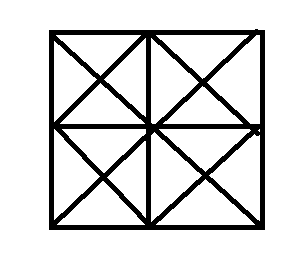74422 1

## Q: In the following questions, count the number of triangles and squares in the given figure.• 1
44 triangles,10 squares
• 2
14 triangles, 16 squares
• 3
27 triangles,6 squares
• 4
36 triangles, 9 squares